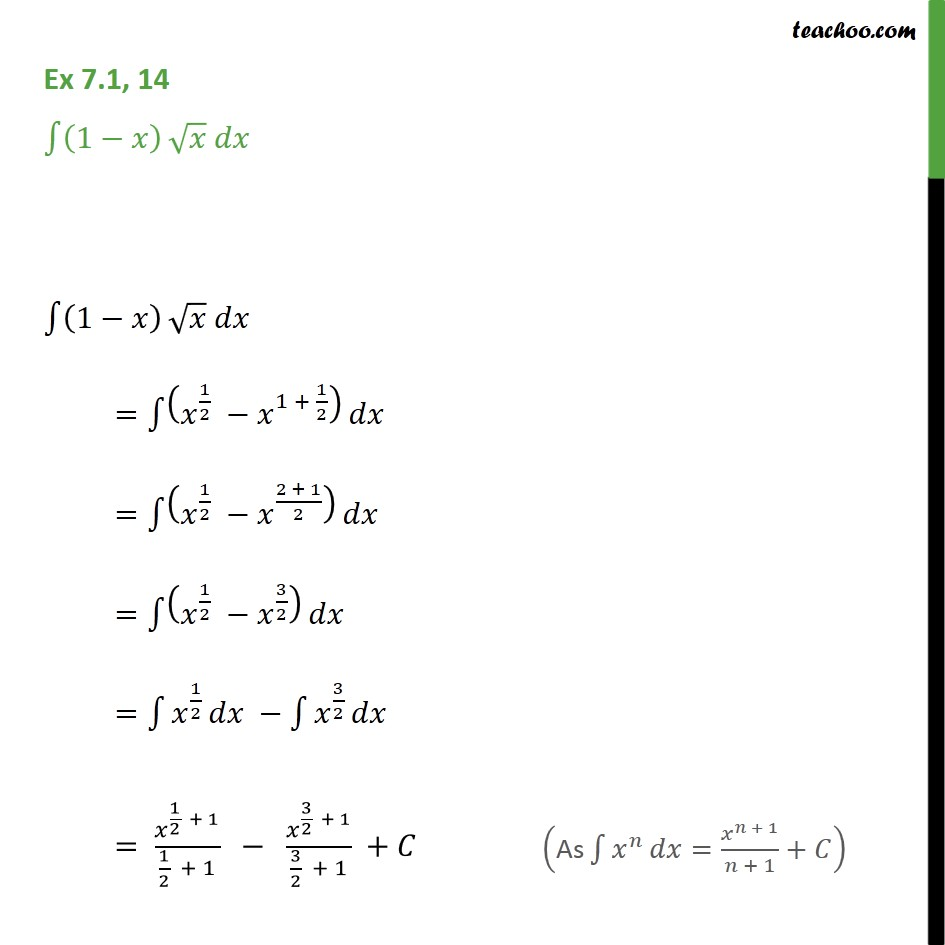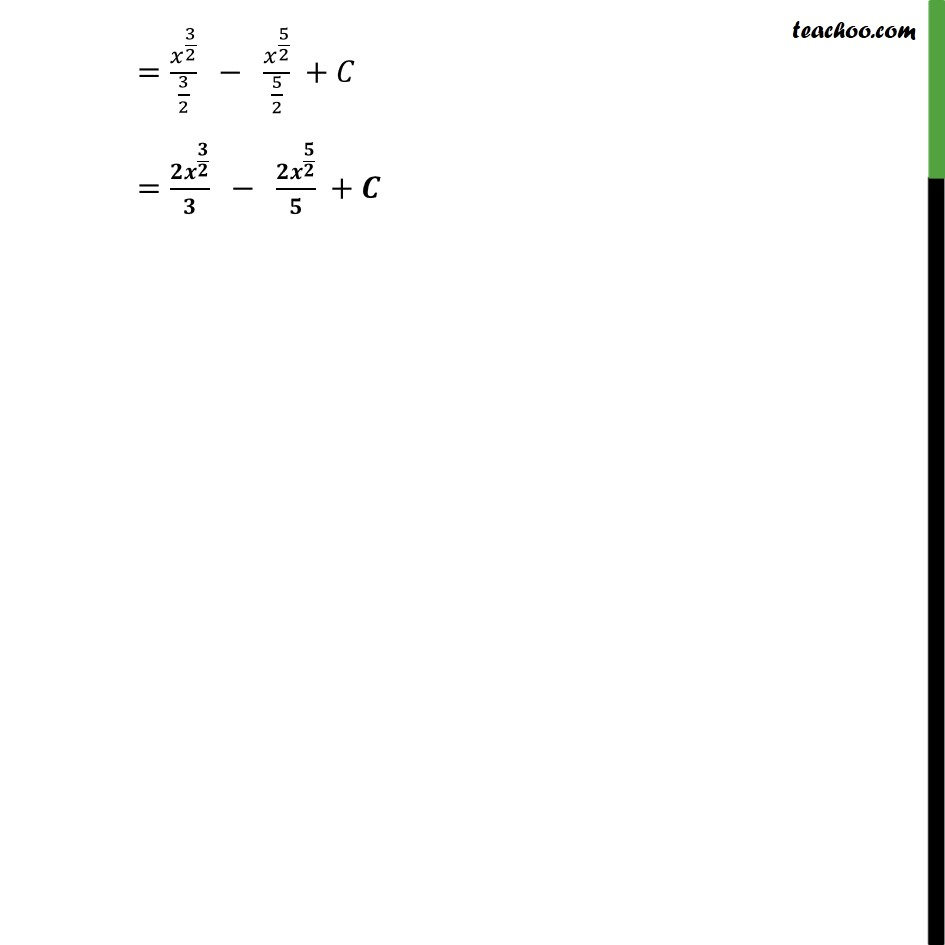Ex 7.1

Chapter 7 Class 12 Integrals
Serial order wiseGet live Maths 1-on-1 Classs - Class 6 to 12

### Transcript

Ex 7.1, 14 1 1 = 1 2 1 + 1 2 = 1 2 2 + 1 2 = 1 2 3 2 = 1 2 3 2 = 1 2 + 1 1 2 + 1 3 2 + 1 3 2 + 1 + = 3 2 3 2 5 2 5 2 + = +